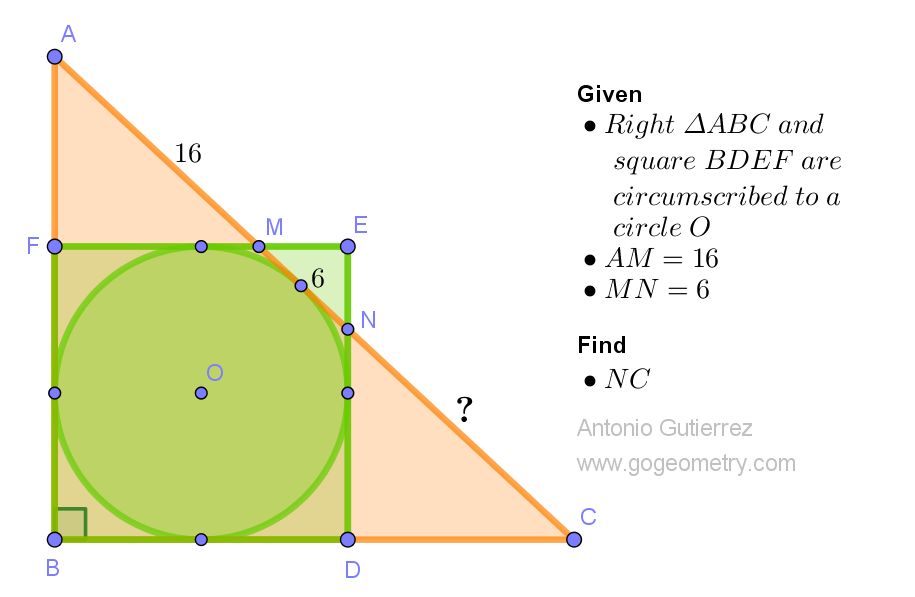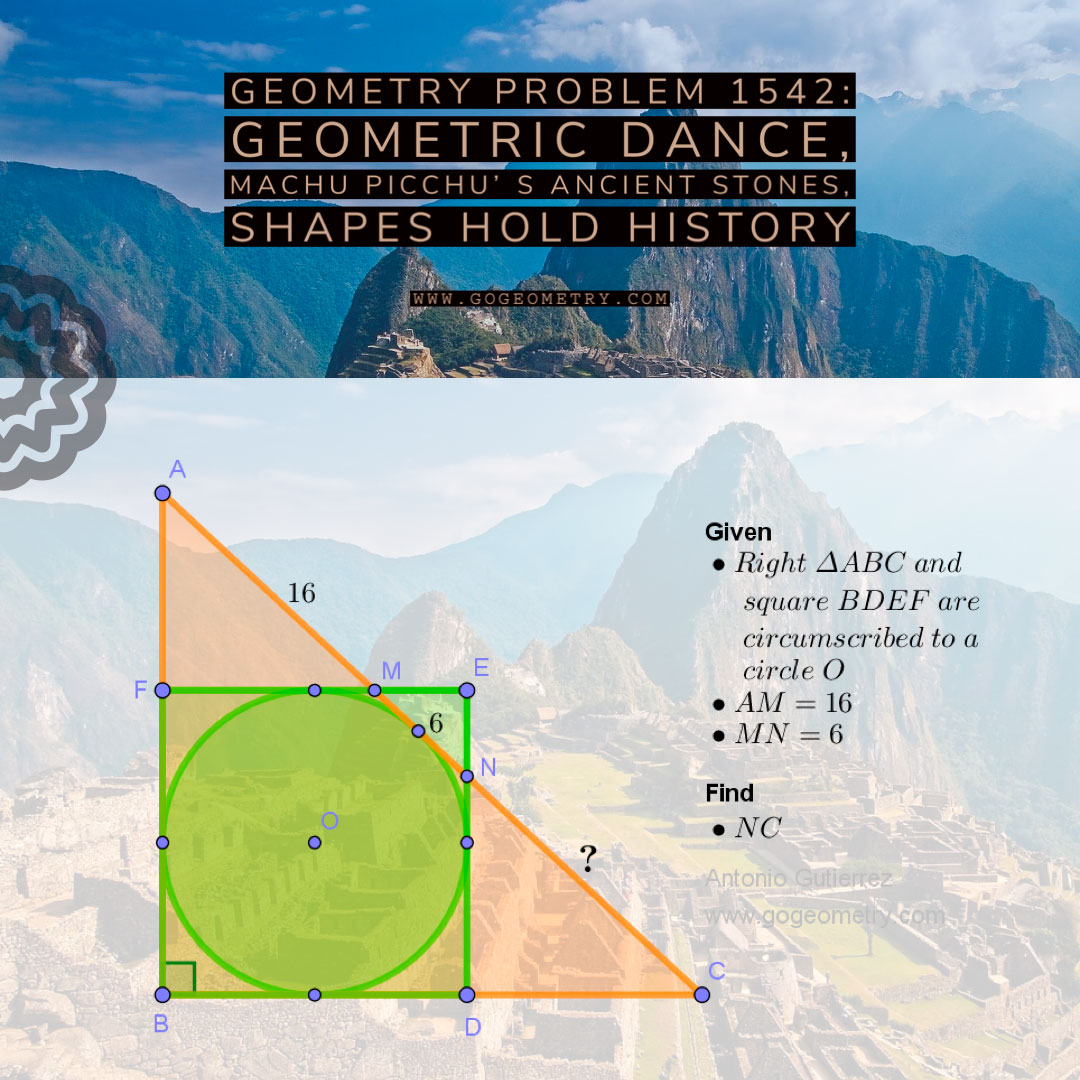# Geometry Problem 1542: Unraveling a Geometric Puzzle with a Circumscribed Right Triangle and Square to the Same Circle for School and College

A right triangle ABC with a right angle at B is circumscribed to a circle with center O, and a square BDEF is also circumscribed to the same circle. Point D lies on line BC, point F lies on line AB, and the lines FE and DE intersect line AC at points M and N respectively. Given that AM = 16 and MN = 6, we need to determine the length of NC.Problem 1542
Triangle, square meet,
Circle's embrace holds their form,
Minds solve geometry.

## Lines and Shadows: Exploring Problem 1542 with Machu Picchu as the Backdrop